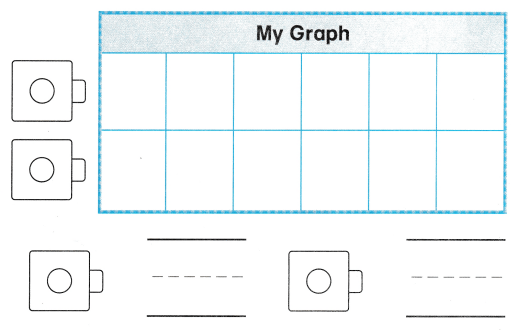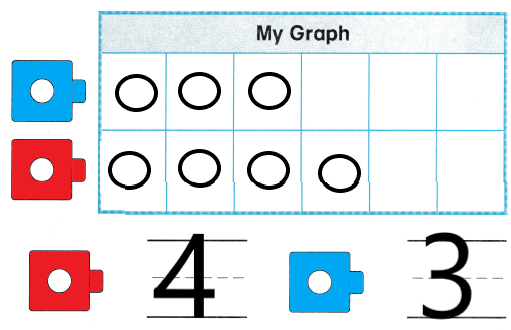# Texas Go Math Kindergarten Unit 5 Assessment Answer Key

Refer to our Texas Go Math Kindergarten Answer Key Pdf to score good marks in the exams. Test yourself by practicing the problems from Texas Go Math Kindergarten Unit 5 Assessment Answer Key.

## Texas Go Math Kindergarten Unit 5 Assessment Answer Key

Vocabulary

Question 1.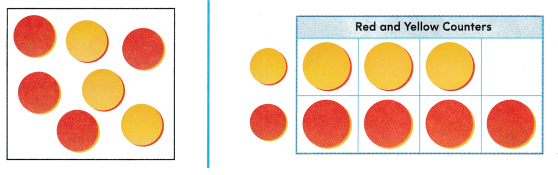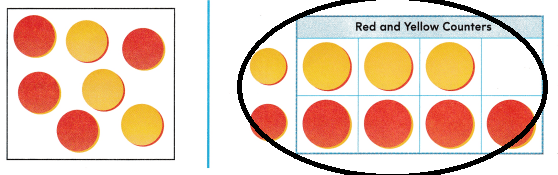Explanation:
The second figure is a graph
so, circled  the second figure.

Concepts and Skills

Question 2.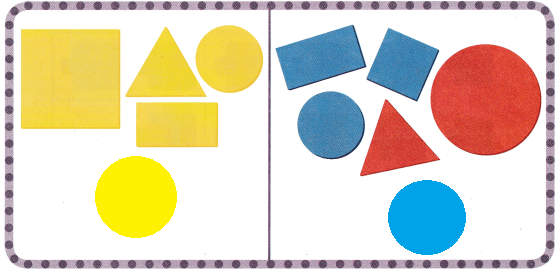Explanation:
Shapes are sorted according to the colors
in first column there is only yellow color and in the second column
there is blue and red colors

Directions
1. Circle the graph. TEKS K.8.B 2. How are the shapes sorted? Draw one more shape in each category. TEKS K.8.A

Question 3.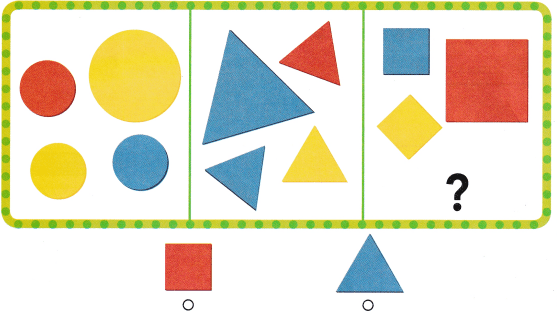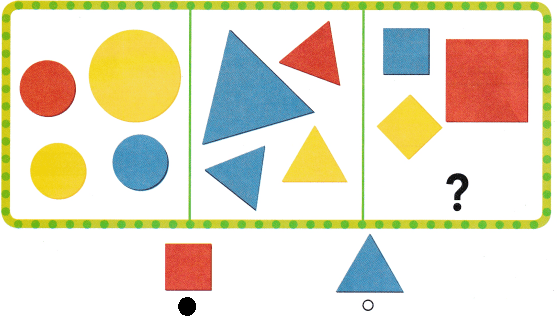Explanation:
There is a square missing in the squares box
so, drawn a square in squares box.

Question 4.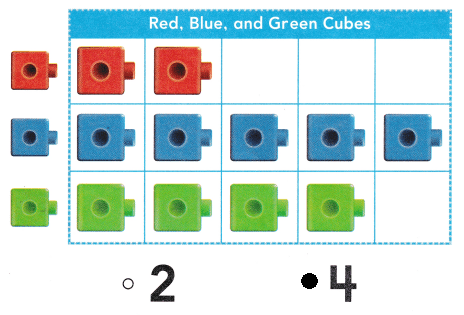Explanation:
There are 4 green cubes
so, marked the number 4.

Directions
Fill in the circle for the correct answer choice. 3. Look at how the shapes are sorted. Which shape belongs in the last category? TEKS K.8.A. 4. Look at the graph. How many green cubes are there? TEKS K.8.C

Texas Test Prep

Question 5.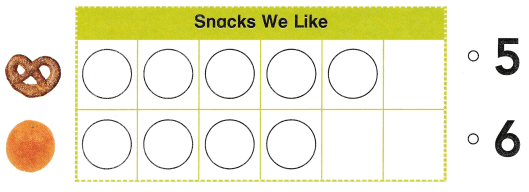Explanation:
5 children like pretzels
so, marked the number 5.

Question 6.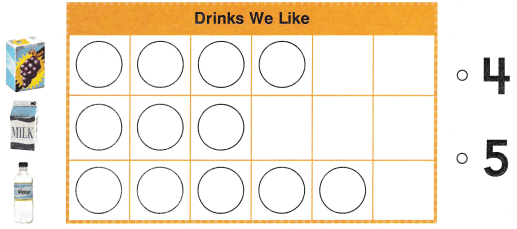Explanation:
5 children like water
so, marked the number 5

Question 7.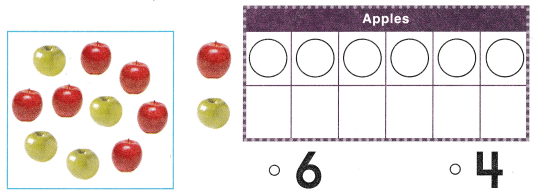Explanation:
There are 6 red apples and 4 green apples
so, marked the number 4.

Directions
5. Look at the picture graph. How many children like pretzels? TEKS K.8.C 6. Look at the picture graph. How many children like water? TEKS K.8.C 7. Collie sorts the apples and makes a graph. How many circles will she draw in the bottom row? TEKS K.8.B